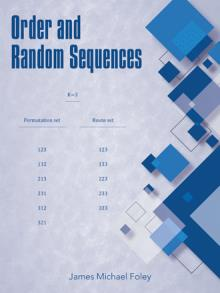Zoom

# Order and Random Sequences

• Also available as: E-Book
• Published: February 2020
• Format: Perfect Bound Softcover(B/W)
• Pages: 250
• Size: 8.25x11
• ISBN: 9781480886971
• Overview
• Free Preview
• Customer Review
• Editorial Review

Consider a set of k elements. Let the elements be the first k positive integers. Consider the permutation set that corresponds to the first k positive integers – and let each element’s order equal that element’s value.

At every line of the permutation set, there exists an element or there exist the elements of the greatest order so far while doing a left-to-right scan. As a consequence, six permutation set properties exist. Expectations and expected values corresponding to the properties are calculated.

Then the order of the elements is generalized. An equal order constraint can be applied so that selected elements of adjacent order are reassigned the lowest order of the elements of adjacent order. Then multiple equal order constraints can be applied concurrently. Then all possible equal order constraints are considered.

Explore order in the context of its effect on random sequences with the calculations and explanations in Order and Random Sequences.